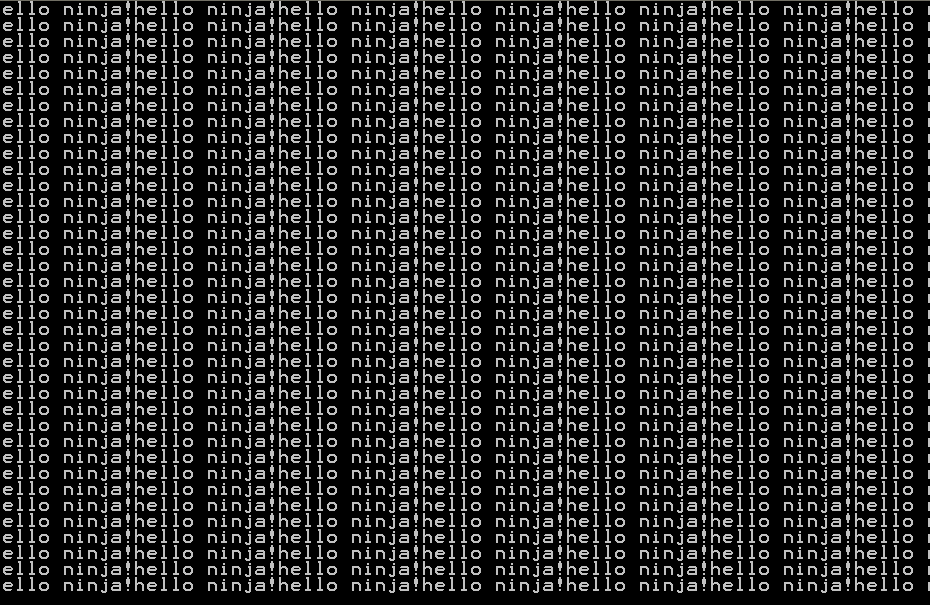# Selection Sort using Recursion

Written by Raza on. Posted in C++

As I mentioned earlier, this time instead of advancing towards another Sorting Algorithm, we would discuss the previous one, that is, the selection sort algorithm in the light of Recursion. So let’s deliberate over recursion first.

## Recursion:

Recursion means to repeat itself. In Computer Programming, recursion is the situation when a particular function calls itself within its own body. Well, it seems weird from here. You might argue that if a function executes its body and then calls itself, that called function would again call itself at the end and this would continue without stopping, in the form of an infinite loop. Suppose we write this code.

```</p>
<p>void Print_Crap ()</p>
<p>{</p>
<p>&nbsp; &nbsp; cout &lt;&lt; "hello ninja!" ;</p>
<p>&nbsp; &nbsp; Print_Crap () ;</p>
<p>}</p>
<p>```

Its result would be something like this, which is a compete wreckage.Having said that, it is pretty important to mention there are certain techniques  and rules to use recursion. Recursion is always performed in a sentinel controlled program. It means that a function would not always call itself; it would call itself only under a specific condition. Otherwise it may become very dangerous as we have seen above.

After a smart little intro on recursion, let me tell you where to use recursion. When you find a portion in your program that is consistently doing the same job over and over again in a loop, you can make use of recursion there. As we talked about selection sort, we saw that in each step we had to find the minimum element in the Array. So instead of the larger loop, we can just call the function again within its body. We would have to make certain changes in the body of the function as well to accommodate recursion. So let’s see what we have to change there.

## Selection Sort :

If we look at that code, there are two loops, one within the first one. The first one decreases the size of the Array by one index in each step. In each step, the second loop finds the minimum value in the Array. So what the outer loop is doing, we can well accomplish it by calling the function again and again at its end. For this purpose, we would have to give the function our integer Array, its constant size and its present size (which gets decreased in each step) as a parameter. We would call the function until the present size becomes one less than the actual constant size of the Array. If you aren’t getting it, you had better read the previous post on selection sort.

The source code of this function along with the main function would look like this.

```</p>
<p>#include &lt;iostream&gt;</p>
<p>using namespace std;</p>
<p>&nbsp;</p>
<p>void Selection_Sort (int arr [], const int elements, int size)</p>
<p>{</p>
<p>&nbsp; &nbsp; int min = size ; &nbsp; &nbsp; &nbsp; &nbsp;//Supposed minimum element at this index</p>
<p>&nbsp; &nbsp; for (int i = size + 1 ; i &lt; elements ; ++i) &nbsp; &nbsp;//starts from the present size + 1 &nbsp; &nbsp; { &nbsp; &nbsp; &nbsp; &nbsp; &nbsp; &nbsp; &nbsp; &nbsp; if (arr [min] &gt; arr [i]) &nbsp; &nbsp; &nbsp; &nbsp;//comparison</p>
<p>&nbsp; &nbsp; &nbsp; &nbsp; {</p>
<p>&nbsp; &nbsp; &nbsp; &nbsp; &nbsp; &nbsp; min = i ;</p>
<p>&nbsp; &nbsp; &nbsp; &nbsp; }</p>
<p>&nbsp; &nbsp; }</p>
<p>&nbsp; &nbsp; if (min != size)</p>
<p>&nbsp; &nbsp; &nbsp; &nbsp; swap (arr [size], arr [min]) ;</p>
<p>&nbsp; &nbsp; ++size ; &nbsp; &nbsp; &nbsp; &nbsp; &nbsp; &nbsp; &nbsp; &nbsp; &nbsp; //size is increased, iterations get decreased</p>
<p>&nbsp; &nbsp; &nbsp;/*The Sentinel Control*/</p>
<p>&nbsp; &nbsp; if (size &lt; (elements - 1)) &nbsp; //if the present size is less than the actual size</p>
<p>&nbsp; &nbsp; &nbsp; &nbsp; Selection_Sort (arr, elements, size) ;</p>
<p>}</p>
<p>int main ()</p>
<p>{</p>
<p>&nbsp; &nbsp; const int ELEMENTS = 10 ; &nbsp; &nbsp; &nbsp; &nbsp; &nbsp; &nbsp; &nbsp; &nbsp; &nbsp; //The constant size of Array</p>
<p>&nbsp; &nbsp; int arr [ELEMENTS] = {6, 2, 3, 7, 9, 1, 4, 10, 8, 5} &nbsp;; &nbsp; &nbsp;//Array Initialized</p>
<p>&nbsp; &nbsp; Selection_Sort (arr, ELEMENTS, 0) &nbsp;; &nbsp; &nbsp; &nbsp;//First Calling of Function &nbsp; &nbsp; &nbsp; &nbsp; &nbsp; &nbsp; &nbsp;</p>
<p>}</p>
<p>```

I hope it made sense. If it didn’t, you had better have a look at the previous article here on Selection Sort Algorithm

Next time we would most probably be exploring Bubble Sort and then the Gnome Sort.

Till then Good Bye!